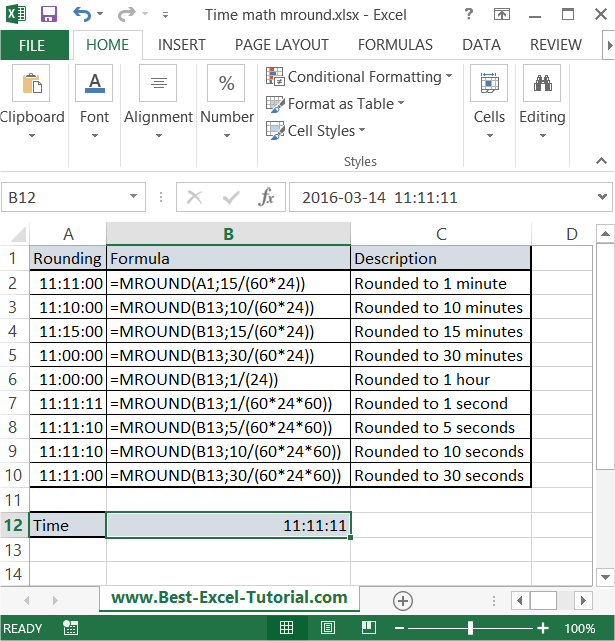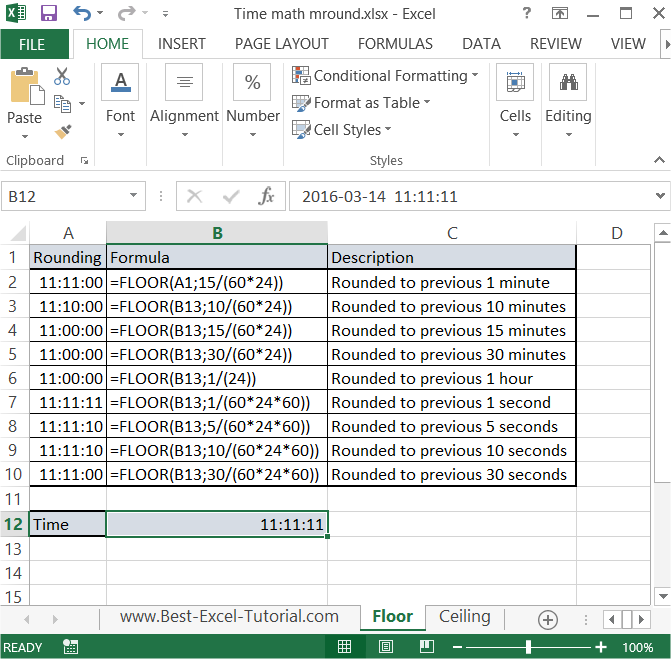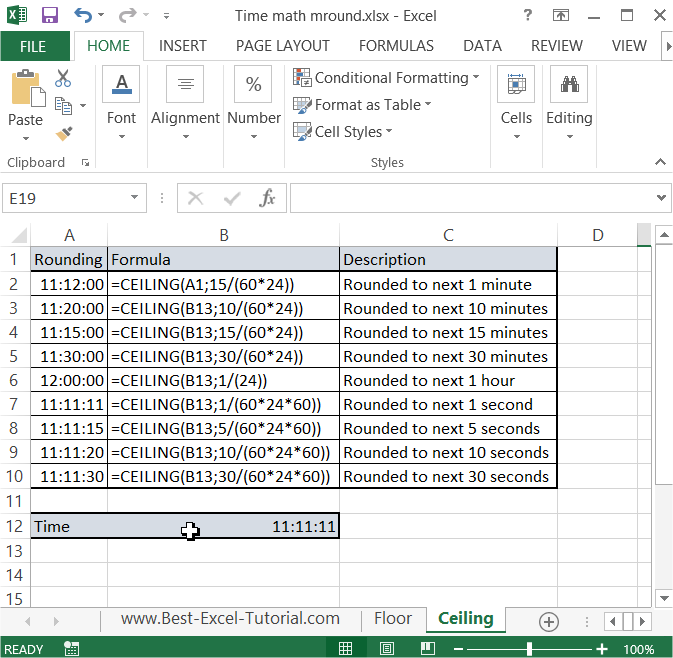#### Round to nearest (time math)

In this Excel tutorial you will learn yourself how to round to nearest time. That kind of time math is useful in spreadsheet where you deal with time.

To round time in Excel just use MROUND function. Syntax is: =MROUND(number, multiple)

Number is the time you want to round.

Multiple is the math you will use to do rounding.

In this example there is a 11:11:11 time in B11 cell. There are several roundings you can do.

## Rounding to minutes

To round to one minute just use =MROUND(B11;1/(60*24)) formula. 60 * 24 is the number of minutes in a day.

To round to 15 minutes change 1 to 15 in formula as follows =MROUND(B11;15/(60*24)) formula.

That’s easy way to round to minutes in Excel.

## Rounding to hours

To round to one hour just use =MROUND(B11;1/(24)) formula. 24 is the number of hours in a day.

## Rounding to seconds

To round to one second just use =MROUND(B11;1/(24*60*60)) formula. 24 * 60 * 60 is the number of seconds in a day.

To round to 15 seconds just use =MROUND(B11;15/(24*60*60)) formula.## Rounding to previous time

To round to previous minute/second/hour just use another function. Instead of MROUND use FLOOR function. Syntax is the same.

Just take a look at below picture and everything should be easy.## Rounding to next time

You can also round to next time. Use CEILING function. Syntax the same.## Rounding to nearest 5

Your task is to round a number in A1 cell to nearest 5. Excel can do that!

### Mround function

The main function which does that is MROUND.

You can use that formula:

=MROUND(A1,5)

### Round function

Another method to round a number to the nearest multiple of 5 in Excel is to use the ROUND function in combination with simple arithmetic.

The same does ROUND function with this formula:

=ROUND(A1/5,0)*5

The formula works by dividing the value in A1 by 5, rounding the result to the nearest whole number using the ROUND function, and then multiplying the result by 5. This effectively rounds the value in A1 to the nearest multiple of 5.

The result in cell B1 would be 25, which is the nearest multiple of 5 to 23.46.

Note that if the value you want to round is exactly halfway between two multiples of 5, this method will also round up to the nearest even multiple. For example, if you use this formula to round 2.5, the result will be 0 (not 5). If you want to round to the nearest odd multiple, you can add or subtract 2.5 from the result before multiplying by 5.

### Alternative methods

If you prefer other function there are two more: CEILING and FLOOR. They work the same such as MROUND but CEILING round up and FLOOR round down.

=CEILING(A1,5)

=FLOOR(A1,5)

Compare these roundings and do some more examples on your own. There are much more possibilities of rounding in Excel.